# ICSE Class 10 Chemistry Question Paper Solution 2019

In board exams few questions from previous years are expected to be asked in the exam every year. So, it’s crucial that while preparing for the exam, students must solve previous years’ question papers. Hence, to help students in their exam preparation we have provided the ICSE Class 10 Chemistry Question Paper 2019 along with the Solutions. Going through this paper solution pdf will give students a new way of approaching the questions during the exam.

The ICSE Class 10 Chemistry 2019 exam was conducted on 22nd March 2019. The exam started at 11am and students were allotted 2 hours of time duration to finish the paper. Students can download the ICSE Class 10 Chemistry Question Paper Solution 2019 PDF from the link below.

Students can have a look at the ICSE Class 10 Chemistry Question Paper Solution 2019 below: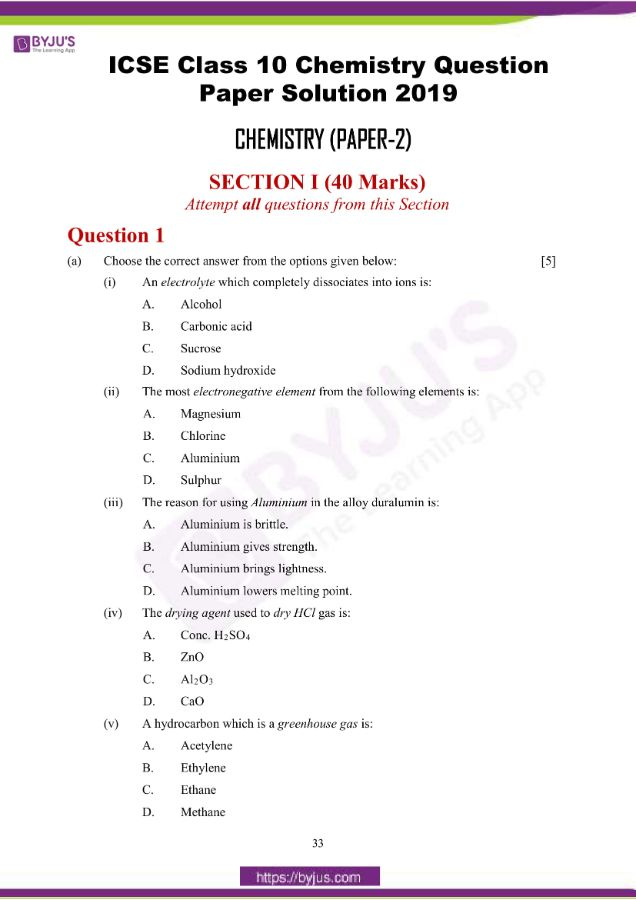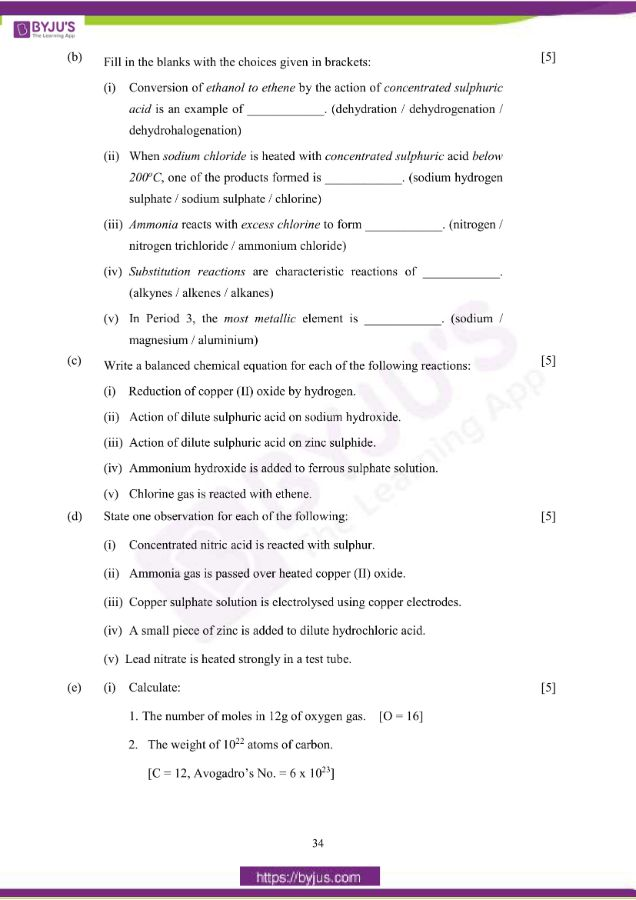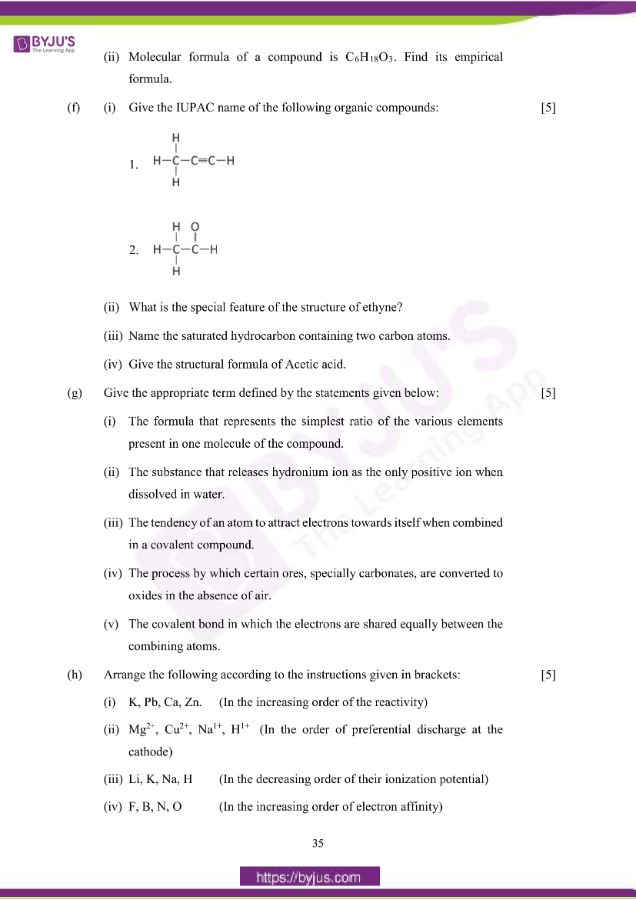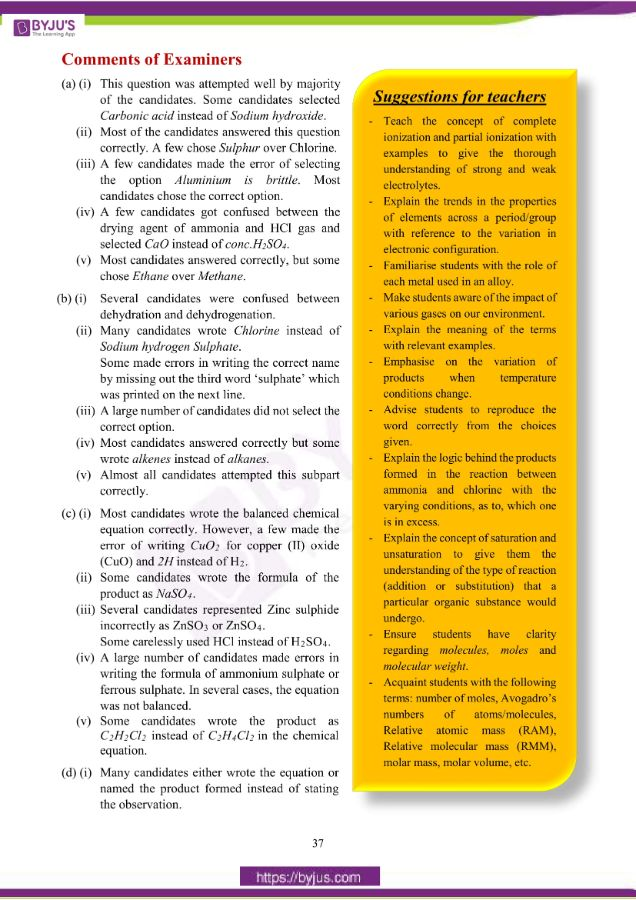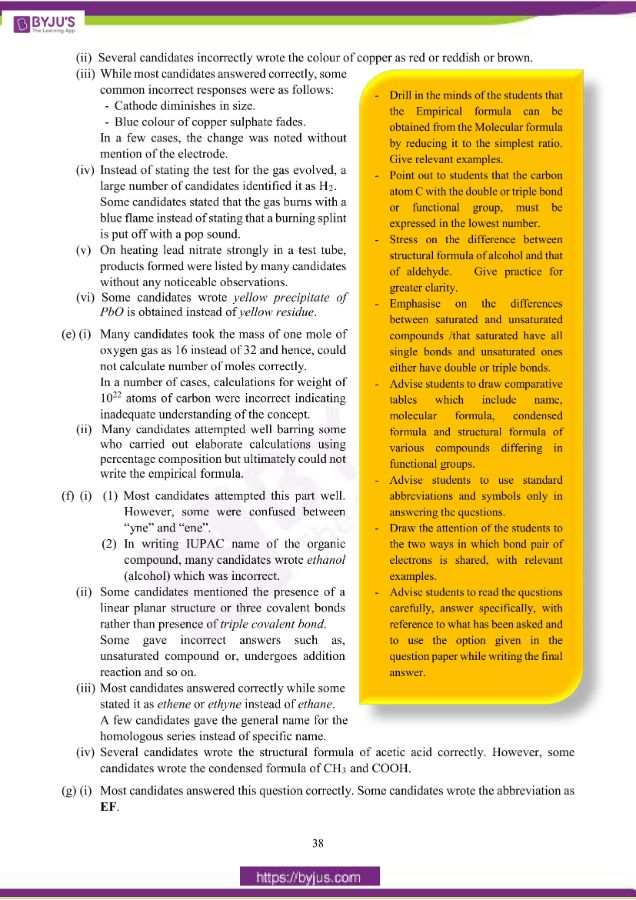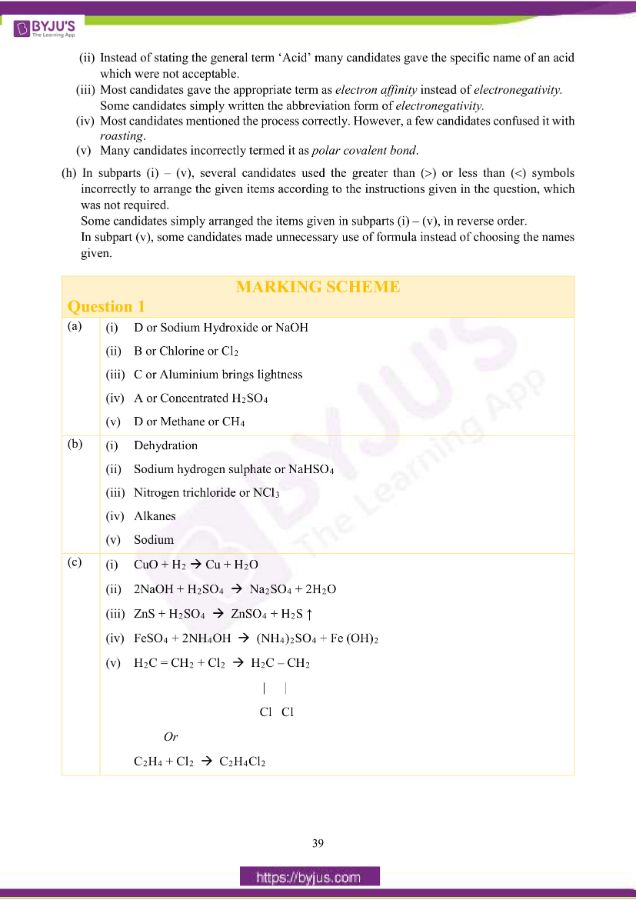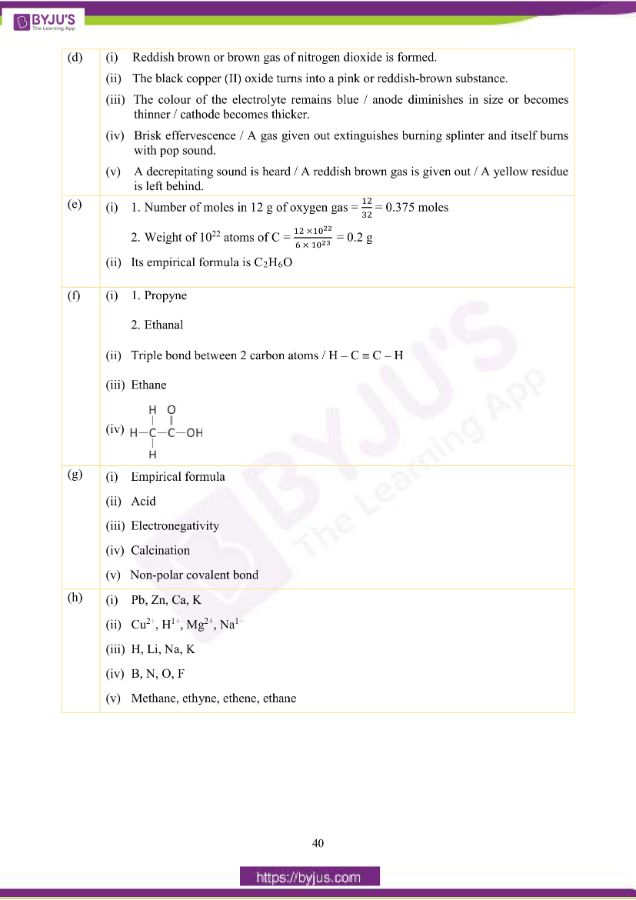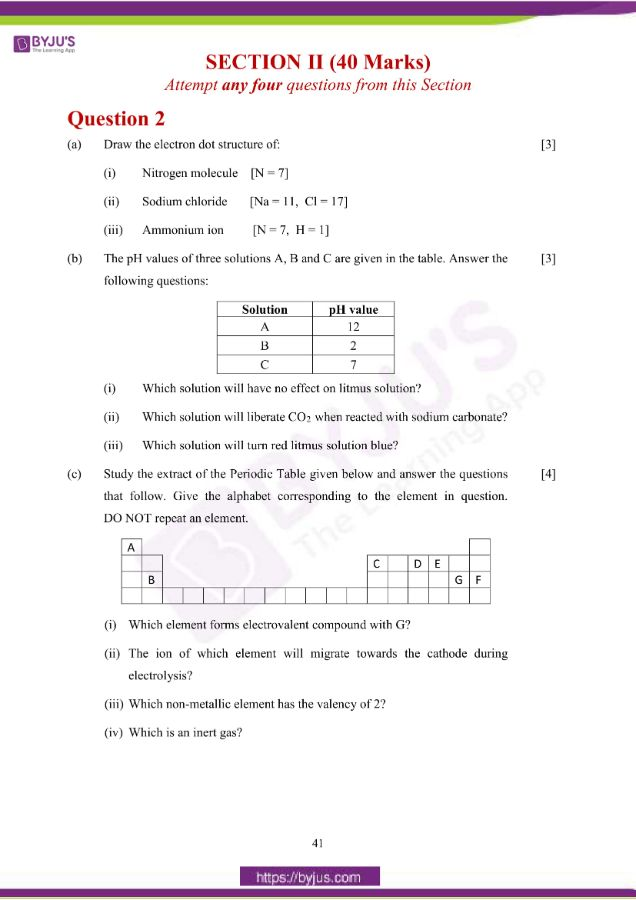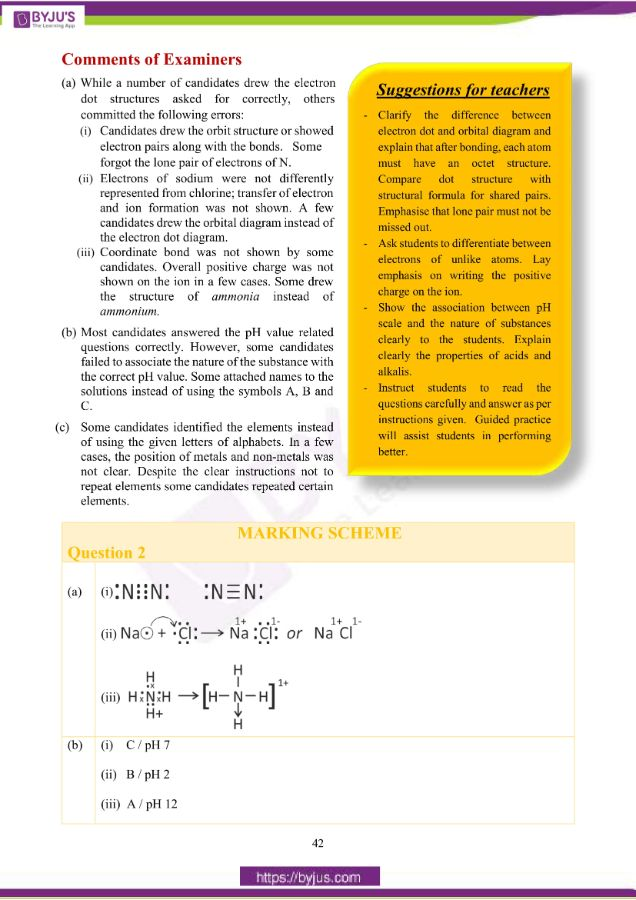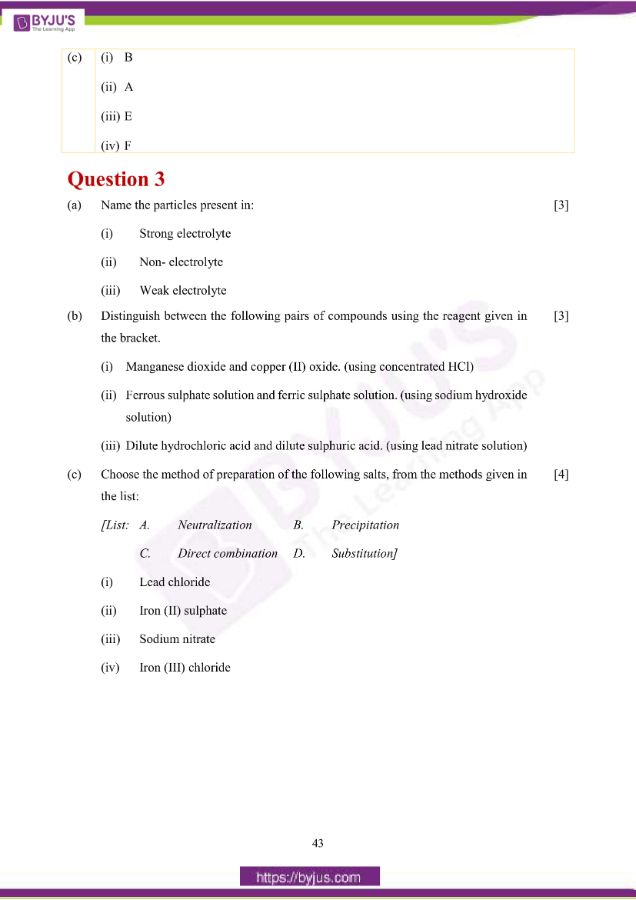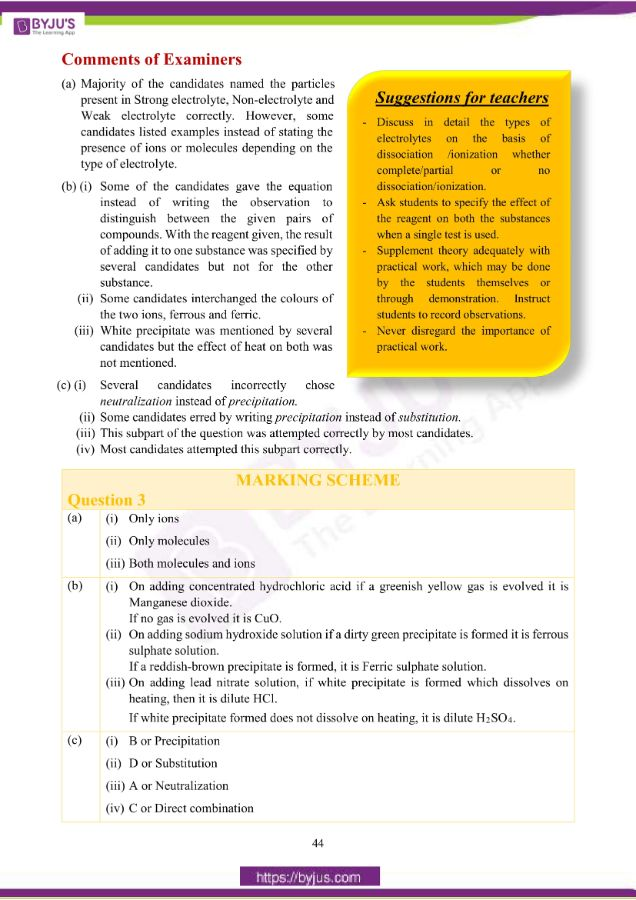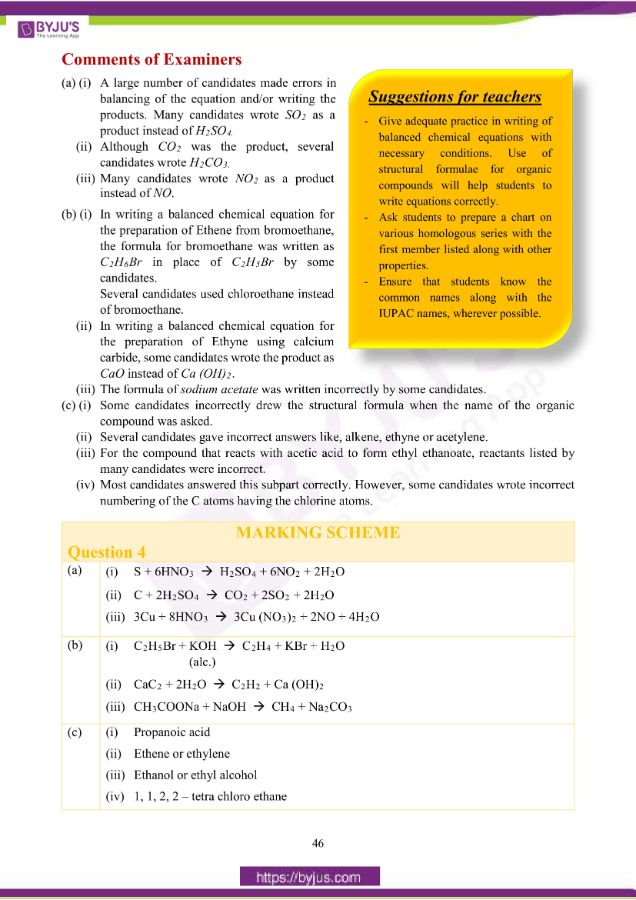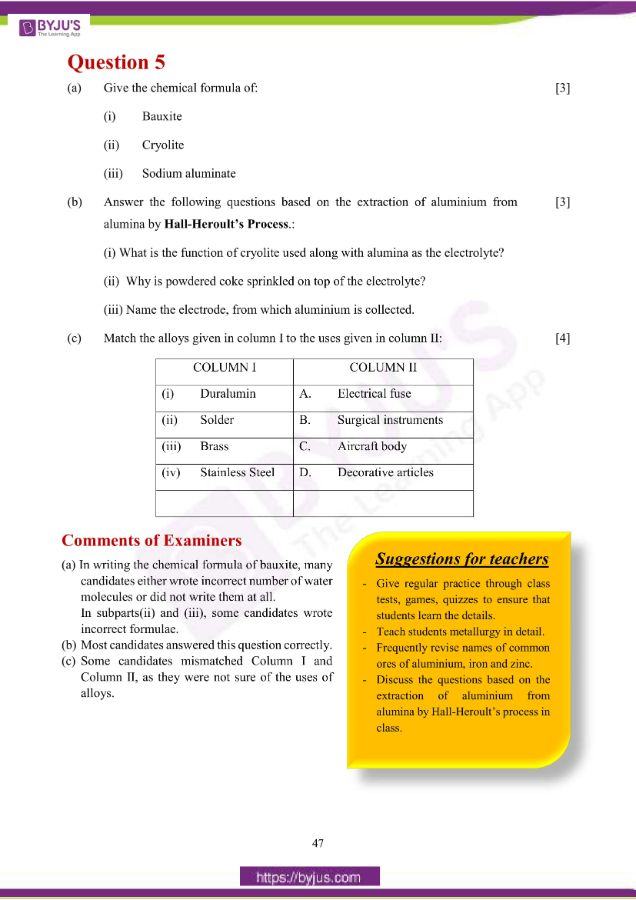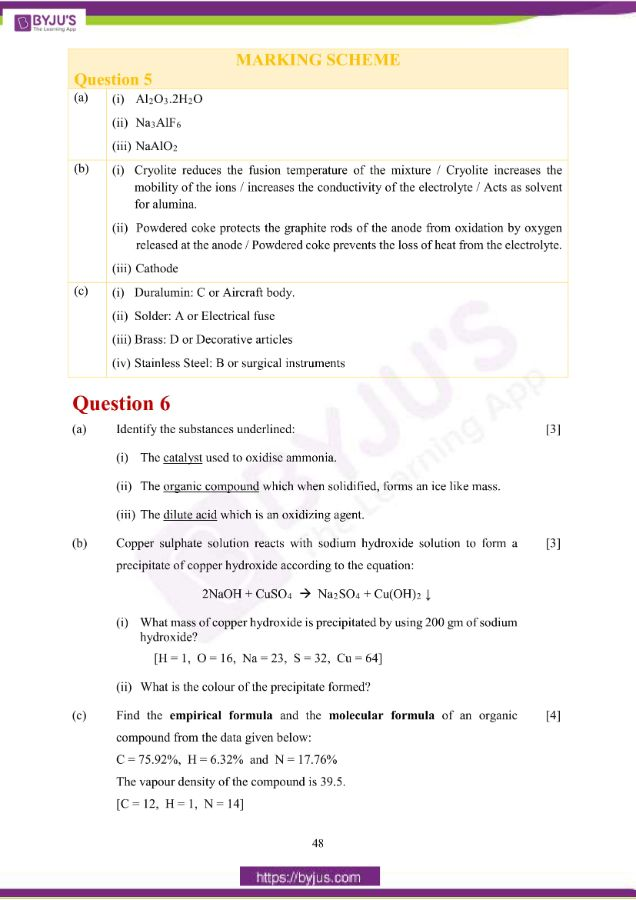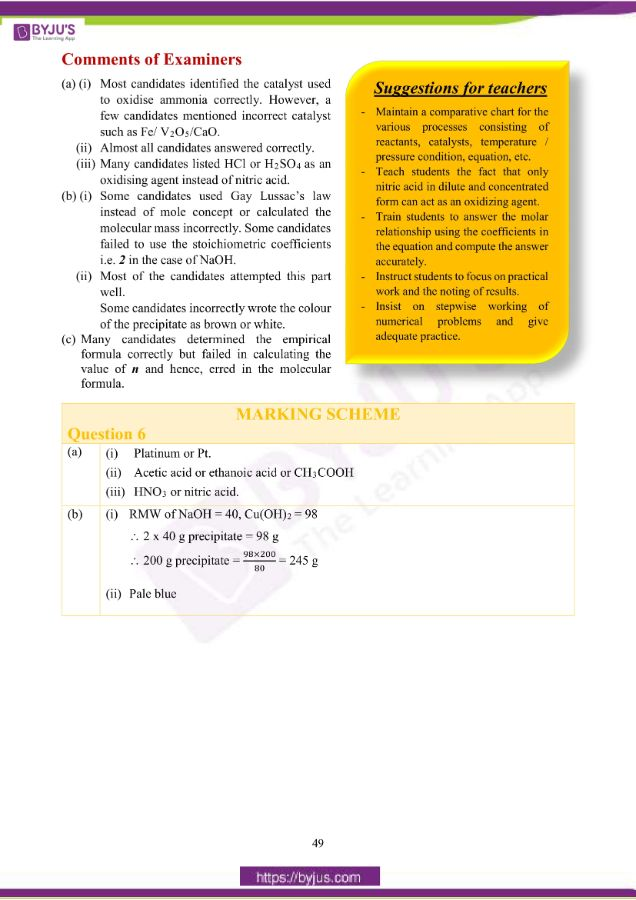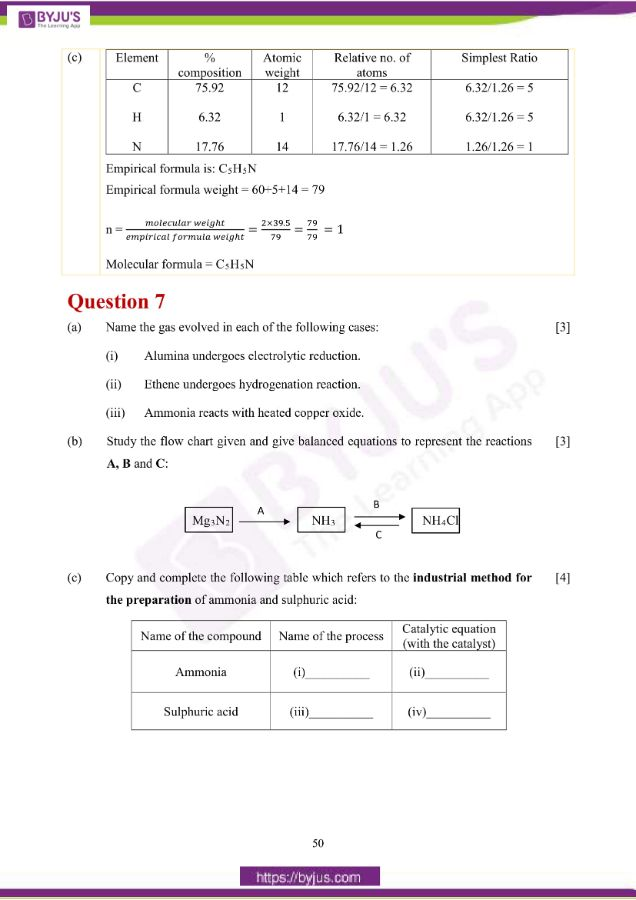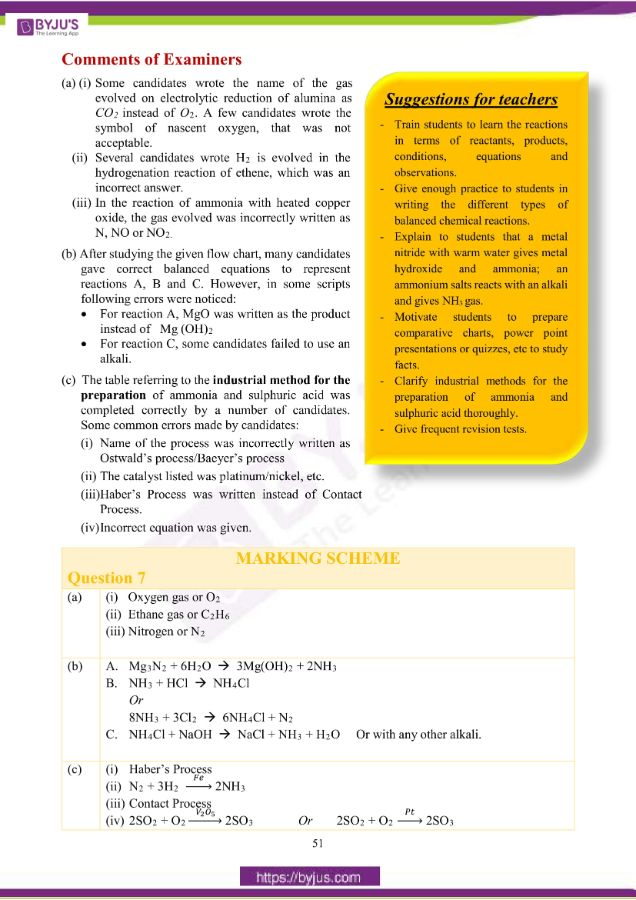## ICSE Class 10 Chemistry (Paper 2) Question Paper 2019 With Solution

### Question 1:

(a) Choose the correct answer from the options given below:

(i) An electrolyte which completely dissociates into ions is:

A. Alcohol

B. Carbonic acid

C. Sucrose

D. Sodium hydroxide

(ii) The most electronegative element from the following elements is:

A. Magnesium

B. Chlorine

C. Aluminium

D. Sulphur

(iii) The reason for using Aluminium in the alloy duralumin is:

A. Aluminium is brittle.

B. Aluminium gives strength.

C. Aluminium brings lightness.

D. Aluminium lowers melting point.

(iv) The drying agent used to dry HCl gas is:

A. Conc. H2SO4

B. ZnO

C. Al2O3

D. CaO

(v) A hydrocarbon which is a greenhouse gas is:

A. Acetylene

B. Ethylene

C. Ethane

D. Methane

(b) Fill in the blanks with the choices given in brackets:

(i) Conversion of ethanol to ethene by the action of concentrated sulphuric acid is an example of ____________. (dehydration / dehydrogenation / dehydrohalogenation)

(ii) When sodium chloride is heated with concentrated sulphuric acid below 200oC, one of the products formed is ____________. (sodium hydrogen sulphate / sodium sulphate / chlorine)

(iii) Ammonia reacts with excess chlorine to form ____________. (nitrogen / nitrogen trichloride / ammonium chloride)

(iv) Substitution reactions are characteristic reactions of ____________. (alkynes / alkenes / alkanes)

(v) In Period 3, the most metallic element is ____________. (sodium / magnesium / aluminium)

(c) Write a balanced chemical equation for each of the following reactions:

(i) Reduction of copper (II) oxide by hydrogen.

(ii) Action of dilute sulphuric acid on sodium hydroxide.

(iii) Action of dilute sulphuric acid on zinc sulphide.

(iv) Ammonium hydroxide is added to ferrous sulphate solution.

(v) Chlorine gas is reacted with ethene.

(d) State one observation for each of the following:

(i) Concentrated nitric acid is reacted with sulphur.

(ii) Ammonia gas is passed over heated copper (II) oxide.

(iii) Copper sulphate solution is electrolysed using copper electrodes.

(iv) A small piece of zinc is added to dilute hydrochloric acid.

(v) Lead nitrate is heated strongly in a test tube.

(e) (i) Calculate:

1. The number of moles in 12g of oxygen gas. [O = 16]

2. The weight of 1022 atoms of carbon.

[C = 12, Avogadro’s No. = 6 x 1023]

(ii) Molecular formula of a compound is C6H18O3. Find its empirical

Formula.

(f) (i) Give the IUPAC name of the following organic compounds: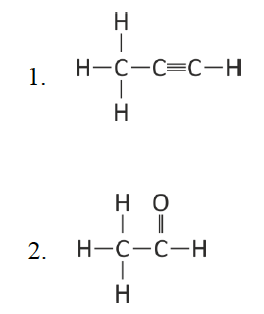(ii) What is the special feature of the structure of ethyne?

(iii) Name the saturated hydrocarbon containing two carbon atoms.

(iv) Give the structural formula of Acetic acid.

(g) Give the appropriate term defined by the statements given below:

(i) The formula that represents the simplest ratio of the various elements present in one molecule of the compound.

(ii) The substance that releases hydronium ion as the only positive ion when dissolved in water.

(iii) The tendency of an atom to attract electrons towards itself when combined in a covalent compound.

(iv) The process by which certain ores, specially carbonates, are converted to oxides in the absence of air.

(v) The covalent bond in which the electrons are shared equally between the

combining atoms.

(h) Arrange the following according to the instructions given in brackets:

(i) K, Pb, Ca, Zn. (In the increasing order of the reactivity)

(ii) Mg2+, Cu2+, Na1+, H1+ (In the order of preferential discharge at the cathode)

(iii) Li, K, Na, H (In the decreasing order of their ionization potential)

(iv) F, B, N, O (In the increasing order of electron affinity)

(v) Ethane, methane, ethene, ethyne. (In the increasing order of the molecular

weight) [H = 1, C = 12]

(a) (i) D or Sodium Hydroxide or NaOH

(ii) B or Chlorine or Cl2

(iii) C or Aluminium brings lightness

(iv) A or Concentrated H2SO4

(v) D or Methane or CH4

(b) (i) Dehydration

(ii) Sodium hydrogen sulphate or NaHSO4

(iii) Nitrogen trichloride or NCl3

(iv) Alkanes

(v) Sodium

(c)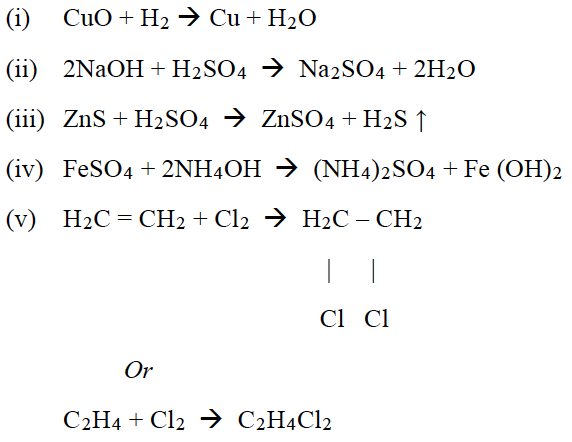(d) (i) Reddish brown or brown gas of nitrogen dioxide is formed.

(ii) The black copper (II) oxide turns into a pink or reddish-brown substance.

(iii) The colour of the electrolyte remains blue / anode diminishes in size or becomes

thinner / cathode becomes thicker.

(iv) Brisk effervescence / A gas given out extinguishes burning splinter and itself burns

with pop sound.

(v) A decrepitating sound is heard / A reddish brown gas is given out / A yellow residue

is left behind.

(e) (i)

1. Number of moles in 12 g of oxygen gas = 12/32 = 0.375 moles

2. Weight of 1022 atoms of C = 12 × 1022 / 6 × 1023 = 0.2 g

(ii) Its empirical formula is C2H6O

(i) 1. Propyne

2. Ethanal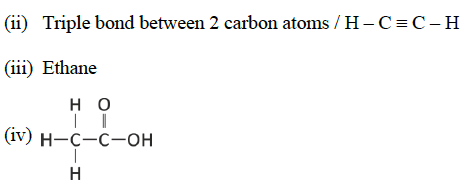(g) (i) Empirical formula

(ii) Acid

(iii) Electronegativity

(iv) Calcination

(v) Non-polar covalent bond

(h)

(i) Pb, Zn, Ca, K

(ii) Cu2+, H1+, Mg2+, Na1+

(iii) H, Li, Na, K

(iv) B, N, O, F

(v) Methane, ethyne, ethene, ethane

### Question 2:

(a) Draw the electron dot structure of:

(i) Nitrogen molecule [N = 7]

(ii) Sodium chloride [Na = 11, Cl = 17]

(iii) Ammonium ion [N = 7, H = 1]

(b) The pH values of three solutions A, B and C are given in the table. Answer the following questions:

 Solution pH value A 12 B 2 C 7

(i) Which solution will have no effect on litmus solution?

(ii) Which solution will liberate CO2 when reacted with sodium carbonate?

(iii) Which solution will turn red litmus solution blue?

(c) Study the extract of the Periodic Table given below and answer the questions that follow. Give the alphabet corresponding to the element in question. DO NOT repeat an element.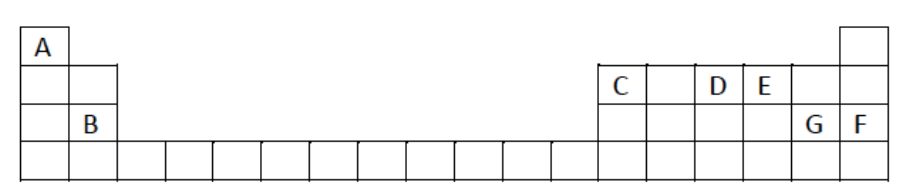(i) Which element forms electrovalent compound with G?

(ii) The ion of which element will migrate towards the cathode during electrolysis?

(iii) Which non-metallic element has the valency of 2?

(iv) Which is an inert gas?

(a)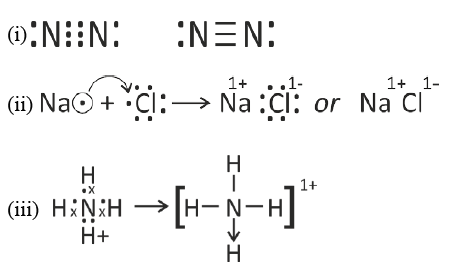(b) (i) C / pH 7

(ii) B / pH 2

(iii) A / pH 12

(c) (i) B

(ii) A

(iii) E

(iv) F

### Question 3:

(a) Name the particles present in:

(i) Strong electrolyte

(ii) Non- electrolyte

(iii) Weak electrolyte

(b) Distinguish between the following pairs of compounds using the reagent given in

the bracket.

(i) Manganese dioxide and copper (II) oxide. (using concentrated HCl)

(ii) Ferrous sulphate solution and ferric sulphate solution. (using sodium hydroxide solution)

(iii) Dilute hydrochloric acid and dilute sulphuric acid. (using lead nitrate solution)

(c) Choose the method of preparation of the following salts, from the methods given in

the list:

[List: A. Neutralization

B. Precipitation

C. Direct combination

D. Substitution]

(ii) Iron (II) sulphate

(iii) Sodium nitrate

(iv) Iron (III) chloride

(a) (i) Only ions

(ii) Only molecules

(iii) Both molecules and ions

(b) (i) On adding concentrated hydrochloric acid if a greenish yellow gas is evolved it is Manganese dioxide.

If no gas is evolved it is CuO.

(ii) On adding sodium hydroxide solution if a dirty green precipitate is formed it is ferrous

sulphate solution.

If a reddish-brown precipitate is formed, it is Ferric sulphate solution.

(iii) On adding lead nitrate solution, if white precipitate is formed which dissolves on heating, then it is dilute HCl.

If white precipitate formed does not dissolve on heating, it is dilute H2SO4.

(c) (i) B or Precipitation

(ii) D or Substitution

(iii) A or Neutralization

(iv) C or Direct combination

### Question 4:

(a) Complete the following equations:

(i) S + conc. HNO3

(ii) C + conc. H2SO4

(iii) Cu + dil. HNO3

(b) Write a balanced chemical equation for the preparation of:

(i) Ethene from bromoethane

(ii) Ethyne using calcium carbide

(iii) Methane from sodium acetate.

(c) Name the following organic compounds:

(i) The compound with 3 carbon atoms whose functional group is a carboxyl.

(ii) The first homologue whose general formula is CnH2n.

(iii) The compound that reacts with acetic acid to form ethyl ethanoate.

(iv) The compound formed by complete chlorination of ethyne

(a) (i) S + 6HNO3 → H2SO4 + 6NO2 + 2H2O

(ii) C + 2H2SO4 → CO2 + 2SO2 + 2H2O

(iii) 3Cu + 8HNO3 → 3Cu (NO3)2 + 2NO + 4H2O

(b)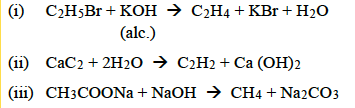(c) (i) Propanoic acid

(ii) Ethene or ethylene

(iii) Ethanol or ethyl alcohol

(iv) 1, 1, 2, 2 – tetra chloro ethane

### Question 5:

(a) Give the chemical formula of:

(i) Bauxite

(ii) Cryolite

(iii) Sodium aluminate

(b) Answer the following questions based on the extraction of aluminium from

alumina by Hall-Heroult’s Process.

(i) What is the function of cryolite used along with alumina as the electrolyte?

(ii) Why is powdered coke sprinkled on top of the electrolyte?

(iii) Name the electrode, from which aluminium is collected.

(c) Match the alloys given in column I to the uses given in column II:

 Column I Column II (i) Duralumin A. Electrical fuse (ii) Solder B. Surgical instruments (iii) Brass C. Aircraft body (iv) Stainless Steel D. Decorative articles

(a) (i) Al2O3.2H2O

(ii) Na3AlF6

(iii) NaAlO2

(b) (i) Cryolite reduces the fusion temperature of the mixture / Cryolite increases the

mobility of the ions / increases the conductivity of the electrolyte / Acts as solvent

for alumina.

(ii) Powdered coke protects the graphite rods of the anode from oxidation by oxygen

released at the anode / Powdered coke prevents the loss of heat from the electrolyte.

(iii) Cathode

(c) (i) Duralumin: C or Aircraft body.

(ii) Solder: A or Electrical fuse

(iii) Brass: D or Decorative articles

(iv) Stainless Steel: B or surgical instruments

### Question 6:

(a) Identify the substances underlined:

(i) The catalyst used to oxidise ammonia.

(ii) The organic compound which when solidified, forms an ice like mass.

(iii) The dilute acid which is an oxidizing agent.

(b) Copper sulphate solution reacts with sodium hydroxide solution to form a

precipitate of copper hydroxide according to the equation:

2NaOH + CuSO4 → Na2SO4 + Cu(OH)2

(i) What mass of copper hydroxide is precipitated by using 200 gm of sodium

hydroxide?

[H = 1, O = 16, Na = 23, S = 32, Cu = 64]

(ii) What is the colour of the precipitate formed?

(c) Find the empirical formula and the molecular formula of an organic

compound from the data given below:

C = 75.92%, H = 6.32% and N = 17.76%

The vapour density of the compound is 39.5.

[C = 12, H = 1, N = 14]

(a) (i) Platinum or Pt.

(ii) Acetic acid or ethanoic acid or CH3COOH

(iii) HNO3 or nitric acid

(b) (i) RMW of NaOH = 40, Cu(OH)2 = 98

∴ 2 x 40 g precipitate = 98 g

∴ 200 g precipitate = 98 x 200/80 = 245 g

(ii) Pale blue

(C)

###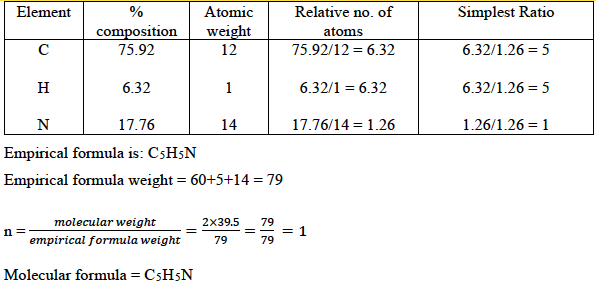Question 7:

(a) Name the gas evolved in each of the following cases:

(i) Alumina undergoes electrolytic reduction.

(ii) Ethene undergoes hydrogenation reaction.

(iii) Ammonia reacts with heated copper oxide.

(b) Study the flow chart given and give balanced equations to represent the reactions

A, B and C: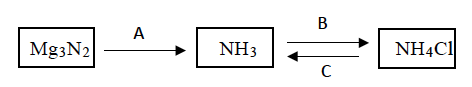(c) Copy and complete the following table which refers to the industrial method for the preparation of ammonia and sulphuric acid: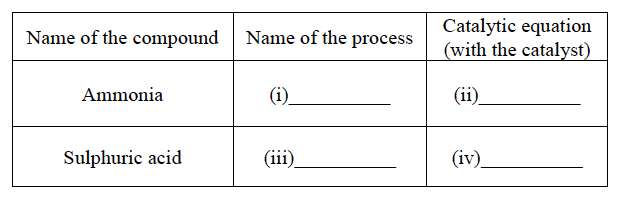(a) (i) Oxygen gas or O2

(ii) Ethane gas or C2H6

(iii) Nitrogen or N2

(b)

A. Mg3N2 + 6H2O → 3Mg(OH)2 + 2NH3

B. NH3 + HCl → NH4Cl

OR

8NH3 + 3Cl2 → 6NH4Cl + N2

C. NH4Cl + NaOH → NaCl + NH3 + H2O Or with any other alkali.

(c)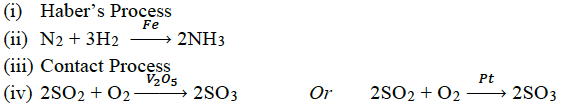We hope this information on “ICSE Class 10 Chemistry Question Papers Solutions 2019” helped students in their exam preparation. To get the year wise ICSE Class 10 Previous Years Question papers along with solutions for other subjects, click here. Keep learning and download BYJU’S App to access interactive study videos.